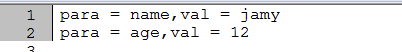# 【PHP】解析PHP中的变量

2018/12/27 22:49

php是一门脚本语言，同时php中的变量类型也是弱语言类型，这和javascript非常相似。笔者在这里说一说PHP中的变量知识点。

# 1. 引用类型变量

<?php
class Ref{
public $val = 100; } function change($v){
$v++; }$Ref = new Ref();
echo $Ref->val."\n";//100 change($Ref->val);
echo $Ref->val."\n";//100 ?> 输出结果为： 100 100 其中符号点(.)表示连接符。 如果把change函数改成如下的形式 function change(&$v){//注意多了&符号
$v++; } 然后输出的结果就是： 100 101 &在上面已经演示过了，&表示引用操作符，顾名思义，被&操作符修饰的变量，表示传递变量的引用。 例如： <?php$foo = "test";

$bar = &$foo;//以引用传递

echo $bar."\n";//test$bar = "hello";

echo $foo."\n";//hello unset($foo);//释放给定的变量

if( isset($bar) ) //检测变量是否已设置 echo "bar is set\n"; else echo "bar is not set\n"; if( isset($foo) ) //检测变量是否已设置
echo "foo is set\n";
else
echo "foo is not set\n";
?>

# 2.可变变量

php中还提供了可变变量这种语法，一个可变变量获取了一个普通变量的值作为这个可变变量的变量名。

<?php
$foo = "hello"; $$foo = "world";$$$foo = "php";

echo $foo."\n";//hello echo $$foo."\n";//world; echo hello."\n";//world echo$$$foo."\n";//php
echo hello."\n";//php
echo $world."\n";//php ?> 下面的案例是摘录自文档中，这里做出如下几点解释。 =>用于给数组赋值时，使用指定（键和值）。 ->用于在访问类的成员字段时，指定要访问的目标字段。 <?php class foo { var$bar = 'I am bar.';
var $arr = array(name=>'jamy.',age=>12); var$r = 'I am r.';
}
$foo = new foo();$bar = 'bar';
$baz = array('foo', 'bar', 'baz', 'quux'); echo$foo->$bar . "\n"; echo$foo->{$baz} . "\n";$start = 'b';
$end = 'ar'; echo$foo->{$start .$end} . "\n";

$arr = 'arr'; echo$foo->{$arr} . "\n"; ?> 输出为： I am bar. I am bar. I am bar. I am r. # 3.超全局变量和局部变量 在PHP中根据变量的作用范围分为全局变量和局部变量。 局部变量：在函数之中定义的变量被称为局部变量。 全局变量：在函数之外声明的变量就是全局变量，若要在函数之中使用全局变量，必须要利用global关键字定义目标变量，或者使用$GLOBLES来指定。

<?php
function myfunc(){
global $foo; echo$foo;//hello
}
$foo = "hello"; myfunc(); ?> 如果使用$GLOBALS同样也可以在函数内访问全局变量。

function myfunc(){
echo $GLOBALS["foo"];//hello }$_GET是通过 URL 参数传递给当前脚本的变量的数组

<?php
$para =$_GET;
foreach (array_keys($para) as$p){
echo("para = ".$p.",val = ".$para[$p]."\n"); } ?> 如果用..../myphpfile.php?name=jamy&age=12 网页源码效果图如下：其余的说明如下：$_SERVER ： 是一个包含了诸如头信息(header)、路径(path)、以及脚本位置(script locations)等等信息的数组。
$_POST：当 HTTP POST 请求的 Content-Type 是 application/x-www-form-urlencoded 或 multipart/form-data 时，会将变量以关联数组形式传入当前脚本。$_GET：通过 URL 参数传递给当前脚本的变量的数组
$_FILES：HTTP文件上传变量，通过 HTTP POST 方式上传到当前脚本的项目的数组。$_SESSION：当前脚本可用 SESSION 变量的数组
$_COOKIE：通过 HTTP Cookies 方式传递给当前脚本的变量的数组。$_REQUEST：request变量,默认情况下包含了 $_GET，$_POST 和 $_COOKIE 的数组。$GLOBALS：包含了全部变量的全局组合数组。变量的名字就是数组的键。

# 4.静态变量

<?php
class foo{
public static $porp = "hello"; } echo foo::$porp;//hello

<?php
$test = function(){ static$count = 0;
echo $count++."\n"; };$test();
$test();$test();

0
1
2

# 5.类实例变量

1.定义类使用关键字class，class后接类名

2.定义构造函数使用__construct，后面接参数。

3.定义访问修饰符，比如：private,public,protected。

4.定义方法使用function关键字，在定义方法时如果不指定访问修饰符，默认为public。

<?php
//user.php
class User {
private $name; private$age;
function __construct( $name,$age ) {
$this->name =$name;
$this->age =$age;
}
function getName() {
return $this->name; } function isAdult() { return$this->age >= 18?"an Adult":"Not an Adult";
}
}

<?php
require "user.php";

$h = new User( "Calvin", 15 ); echo "Hello, " .$h->getName(). "! You are ". $h->isAdult(); ?> <br/> <?php$h = new User( "Chris", 39 );
echo "Hello, " . $h->getName(). "! You are ".$h->isAdult();
?>

http://php.net/

0
0 收藏

### 作者的其它热门文章0 评论
0 收藏
0